﻿ 基于MAS与PSO算法的土地资源决策优化配置研究 Optimized Allocation of Land Resource Based on MAS and PSO Model

Modern Management
Vol.08 No.02(2018), Article ID:24633,13 pages
10.12677/MM.2018.82024

Optimized Allocation of Land Resource Based on MAS and PSO Model

Rongbao Zheng1,2*, Meizhao Chen3, Yaqi Zhang3

1The open Fund of Key Laboratory of Urban Land Resources Monitoring and Simulation, Ministry of Land and Resources, Shenzhen Guangdong

2College of Management, Guangdong University of Technology, Guangzhou Guangdong

3School of Politics and Public Administration, Guangdong University of Foreign Studies, Guangzhou Guangdong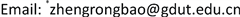Received: Apr. 8th, 2018; accepted: Apr. 21st, 2018; published: Apr. 28th, 2018ABSTRACT

The optimal allocation of land resources is very important to achieve sustainable utilization of land resources, but it is very difficult to realize the harmonization of multi-interest between different subjects. This paper takes the maximization of social, economic and ecological benefits as the goal, and adopts the method of multi-agent system (MAS) and particle swarm optimization (PSO), comprehensively considers the interrelationship between agents, and obtains a number of land resources optimization configuration program, and a comprehensive evaluation of different projects. Results show that: 1) The combination of MAS and PSO can be used to allocate different land use types into different spatial units in the multi-objective constraints in the planning period, so as to realize the optimal allocation of regional land resources. 2) Gray-related method and gray multi-objective decision making method are used to evaluate the different projects, and results show that scheme 2 is most valuable solution, and its value reaches 0.951, so it has been proved to be the optimal scheme.

Keywords:Land Resource, Optimized Allocation, Multi-Agent System, Particle Swarm Optimization, Huangpu District

1国土资源部城市土地资源监测与仿真重点实验室，广东 深圳

2广东工业大学管理学院，广东 广州

3广东外语外贸大学政治与公共管理学院，广东 广州1. 前言

2. 思路与方法

2.1. 典型样区选择——广东省广州市黄埔区为例

2.2. 研究思路

2.3. 土地利用优化模型

2.3.1. 变量设定

2.3.2. 目标函数

1) 经济效益。主要通过实现各类土地利用在经济方面以低成本高收益为主的优化配置。因此，选取土地利用经济总产出最大化区域和最小化区域所转化的成本作为经济效益优化目标结果。

$Max{F}_{1}=\sum _{k=1}^{k}{W}_{k}{S}_{k}$ (1)

$Min{F}_{2}=\sum _{i=1}^{N}\sum _{j=1}^{M}\sum _{k=1}^{k}{C}_{ijk}{X}_{ijk}$ (2)

2) 社会效益。是指为社会发展所提供的基本社会保障服务，本文以社会基本保障功能作为社会效益目标优化的结果。

$Max{F}_{3}=\sum _{k=1}^{k}{G}_{k}{S}_{k}$ (3)

3) 生态效益。指土地利用优化配置对生态环境友好度和生态服务功能的影响。因而，该目标以对生态环境的兼容性和对生态系统的服务功能的总价值为优化对象。

$Max{F}_{4}=\sum _{k=1}^{k}{P}_{k}{S}_{k}$ (4)

$Min{F}_{5}=\sum _{i=1}^{N}\sum _{j=1}^{M}\sum _{k=1}^{k}\sum _{{i}^{\prime }=1}^{N}\sum _{{j}^{\prime }=1}^{M}\sum _{{k}^{\prime }=1}^{k}{L}_{ij,{i}^{\prime }{j}^{\prime }}{H}_{k,{k}^{\prime }}$ (5)

2.3.3. 约束条件

1) 人口总量是土地承载总人口不应该超过国家规定预期人口数量：

${M}_{1}{S}_{f}+{M}_{2}{S}_{u}\le P$ (6)

2) 土地面积为各种土地利用类型面积之和为研究区域总面积：

$\sum _{k=1}^{K}{S}_{k}=S$ (7)

3) 劳动力资源为不同土地利用类型从事相关职业的劳动力数量：

$\sum {l}_{ak}{S}_{k}\le {L}_{a}$ (8)

$\sum {l}_{ck}{S}_{k}\le {L}_{c}$ (9)

4) 粮食安全

${S}_{g}{f}_{2}{f}_{3}{f}_{4}\ge P{f}_{0}{f}_{1}$ (10)

5) 生态环境

${S}_{e}\cdot c\ge S\cdot r{S}_{e}\cdot c\ge S\cdot r$ (11)

6) 市场经济

${S}_{k1}\ge {S}_{k0}$ (12)

${S}_{w1}\ge {S}_{w0}$ (13)

7) 土地利用公平性

a) 土地利用规划期末的各类土地生态能力总和不得低于基期：

$\left(E{P}_{0}^{k}-E{P}_{C}^{k}\right)×\left({P}_{k}\right)\ge E{P}_{0}^{k}$ (14)

b) 耕地非农化时序约束条件：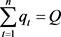(15)

8) 空间布局

a) 空间单元所含至少一种土地类型：

$\sum _{k=1}^{K}{X}_{ijk}=1$ (16)

b) 新增建设用地在空间扩展时的紧凑性：

$\sum _{i,j\in \Omega }{B}_{ijk}\ge 2$ (17)

2.4. 多智能体及决策行为

2.4.1. 上级政府Agent (Agent1)决策

Agent1依据国家政策和区域总体发展目标，凝练区域土地利用战略，并在土地利用规划中分解各项规划指标的限制性因子。本文采用层次分析法对各因子依次赋予权重，并进行多因素综合分析模型，确定112个行政村(社区)占黄埔区的比例，并依据(公式19)确定各行政村的指标。

${F}_{j}=\sum {W}_{ys}\sum {W}_{yz}×{M}_{ij}$ (18)

$Z{B}_{j}={F}_{j}×Z{B}_{sum}$ (19)

2.4.2. 地方政府Agent (Agent2)决策

$ES={\left(\prod _{k=1}^{n}E{S}_{k}\right)}^{t/n}$ (20)

2.4.3. 利用主体Agent(Agent3)决策

1) Agentu合作与竞争算子

a) 竞争算子。当 ${L}_{i,j}$ 满足公式21时，其空间解的位置不变，否则的空间解的位置将按照公式22进行修正，且当 ${L}_{i,j}$ 所在网格的土地类型将调整的与 ${M}_{i,j}$ 保持一致。

$Fit{\left({L}_{i,j}\right)}^{*}\ge Fit{\left({M}_{i,j}\right)}^{*}$ (21)

${l}_{i,j,k}={m}_{i,j,k}+rand\left(-1,1\right)\cdot \left({m}_{i,j,k}-{l}_{i,j,k}\right),\text{\hspace{0.17em}}k-1,\cdots ,n$ (22)

b) 合作算子。在 ${M}_{i,j}$${L}_{i,j}$ 选择随机交叉点，并将交叉点后半部分进行交换，同时在交叉点处进行交叉，从而获得新的Agent。假设 ${M}_{i,j}$${L}_{i,j}$ 在k点进行交叉，而后所产生的两个Agent分支则为：

${{M}^{\prime }}_{I,J}=\left({m}_{i,j,1},{m}_{i,j,2},\cdots ,{{m}^{\prime }}_{i,j,k},{l}_{i,j,k+1},{l}_{i,j,k+2},\cdots ,{l}_{i,j,n}\right)$ (23)

${{L}^{\prime }}_{I,J}=\left({l}_{i,j,1},{l}_{i,j,2},\cdots ,{{l}^{\prime }}_{i,j,k},{m}_{i,j,k+1},{m}_{i,j,k+2},\cdots ,{m}_{i,j,n}\right)$ (24)

${v}_{i,j,k}^{d+1}=w\cdot {v}_{i,j,n}^{d}+{\varphi }_{1}\cdot rand\left(\text{ }\right)\cdot \left({P}_{i,j,n}-{l}_{i,j,n}^{d}\right)+{\varphi }_{2}\cdot rand\left(\text{ }\right)\cdot \left({G}_{i,j,n}-{l}_{i,j,n}^{d}\right)$ (25)

${l}_{i,j,n}^{d+1}={l}_{i,j,n}^{d}+{v}_{i,j,n}^{d}$ (26)

2) Agentu自学性算子

Agentu自学性算子主要用于模型中自适应性最强的Agentu，学习检索的半径为小于5-10个空间单元(T)，并以此为中心，检索产生 $T×T$ 的单元合集，进而对Agentu执行竞争和合作算子，经过不断迭代，该Agentu的自学性将会进一步提高。

3) Agentu适应性算子

${F}_{x}\left({L}_{i,j}\right)=\left\{\begin{array}{l}{\left(N-{Y}_{x}\left({L}_{i,j}\right)\right)}^{2},{Y}_{x}\left({L}_{i,j}\right)>1;\\ k{N}^{2},{Y}_{x}\left({L}_{i,j}\right)=1;\end{array}$ (27)

$Fit\left({L}_{i,j}\right)=\sum _{x=1}^{n}{W}_{x}{F}_{x}\left({L}_{i,j}\right)$ (28)

$Fit{\left({L}_{i,j}\right)}^{*}=Fit\left({L}_{i,j}\right)\cdot {}^{t}S{}_{i,j}^{K}\cdot {}^{t}N{}_{i,j}^{K}\cdot {}^{t}F{}_{i,j}^{K}\cdot V$ (29)

4) Pareto寻优策略

3. 结果与分析

3.1. 模型简化

3.1.1. 上级政府Agent1的决策行为选择

3.1.2. 地方政府Agent2的决策行为选择

3.1.3. 利用主体Agent3的决策行为选择Table 1. Main indicators of land use optimization allocation in Huangpu (hm2)Table 2. Factors of niche-fitness evaluation for land use function zoning

Agent3通过合作、竞争和自学性行为运算进行的空间决策须按照公式29计算Agent3的自适应水平。确定Agentu模型设定目标的观测值以及、 ${}^{t}N{}_{i,j}^{K}{}^{t}N{}_{i,j}^{K}$${}^{t}F{}_{i,j}^{K}{}^{t}F{}_{i,j}^{K}$$VV$${}^{t}F{}_{i,j}^{K}{}^{t}F{}_{i,j}^{K}$$\left(i,j\right)\left(i,j\right)$ 空间单元拟被转化的k土地类型与 $\left(i,j\right)$ 所在功能区兼容性程度越强， ${}^{t}F{}_{i,j}^{K}$ 数值越大，采用Delphi Method求得各种土地利用类型的与功能区的兼容性如表3。Agent3对目标(1)~(5)的观测值所在的经济产出、转换成本、生态功能服务功能价值、生态环境兼容性和社会保障功能总和得到。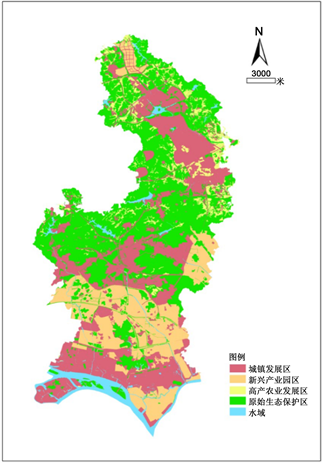Figure 1. Land use function zones of HuangpuTable 3. Compatibility between land use types and function zones

3.2. 结果与分析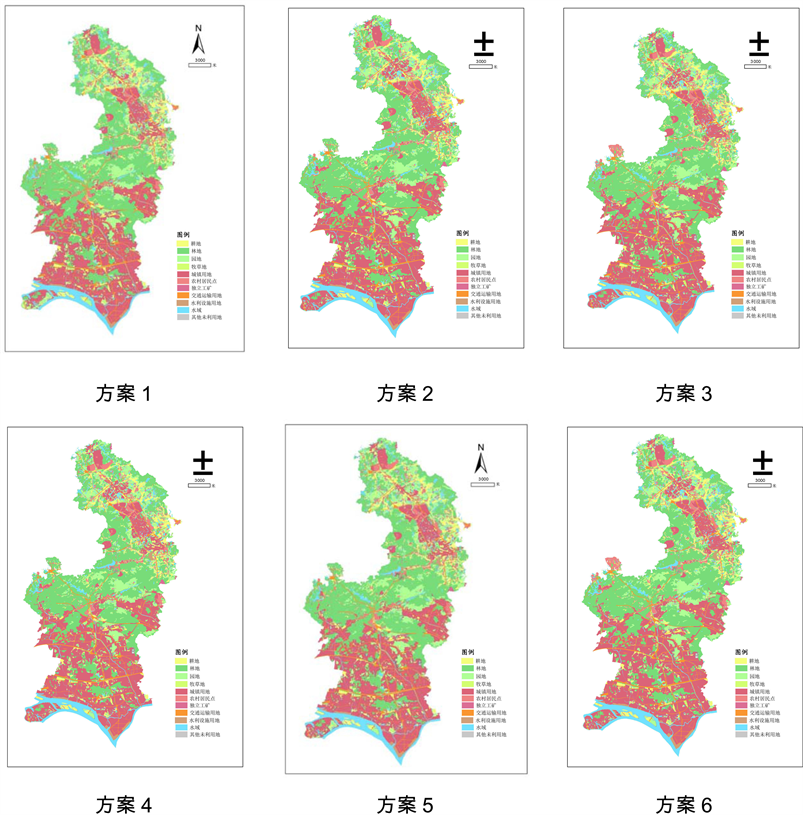Figure 2. Comparison of spatial patterns of alternative sustainable land use optimizationTable 4. Comparison of structures of alternative land use optimization allocation (hm2)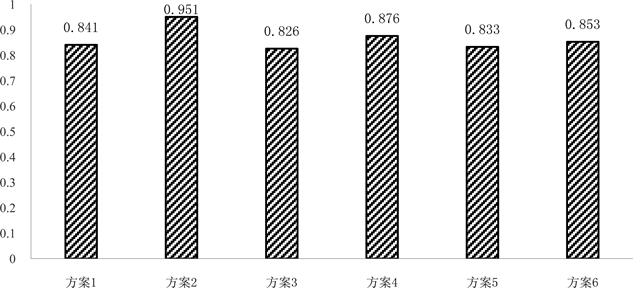Figure 3. Preferred result of sustainable land use optimization allocation in Huangpu

4. 结论与讨论

1) 本文以我国经济快速发展地区广州市黄埔区为例，以土地利用经济、社会和生态效益最大化为目标，定义了三个不同类型的智能体，通过嵌入帕累托最优算法与POS优化算法相结合，构建了不同的土地资源优化配置的决策方案，结果表明该研究思路是基本可行的，为区域土地资源优化配置提供了一种新思路，研究结果可为区域土地资源合理利用提供参考建议。

2) 多智能体系统(MAS)与微粒群算法(PSO)“自下而上”的集群模型在其它领域的应用已日趋成熟，该模型的关键是如何准确地获得各Agents的决策行为及规则，并通过定量化方法进行表达，如果准确确定公式中的参数非常困难，需要开展更多的实验论证。

3) 土地资源的优化配置是一项系统工程，其配置的合理与否关键是要实现社会、经济和生态效益等综合效益的最大化，本文的研究思路中，对社会与经济因素考虑的较多，对环境因素，如地形、河流等考虑较少，对模型运行的多种方案的综合比较可能还不够合理，今后还需要对研究思路与方法作进一步的改进以提高其运行精度。

Optimized Allocation of Land Resource Based on MAS and PSO Model[J]. 现代管理, 2018, 08(02): 192-204. https://doi.org/10.12677/MM.2018.82024

1. 1. 郑荣宝, 董玉祥, 陈梅英. 基于GECM与CA+ANN模型的土地资源优化配置与模拟[J]. 自然资源学报, 2012, 27(1): 1-13.

2. 2. Benabdallah, S. and Wright, J.R. (1992) Multiple Subregion Allocation Models. Journal of Urban Planning and Devel-opment, 118, 24-40. https://doi.org/10.1061/(ASCE)0733-9488(1992)118:1(24)

3. 3. Wang, X.H., Yu, S. and Huang, G.H. (2004) Land Allocation Based on Integrated GIS-Optimization Modeling at a Watershed Level. Landscape and Urban Planning, 66, 61-74. https://doi.org/10.1016/S0169-2046(03)00095-1

4. 4. Ren, F.H. (1997) A Training Model for GIS Application in Land Resource Allocation. ISPRS Photogrammetry and Remote Sensing, 2, 261-265. https://doi.org/10.1016/S0924-2716(97)00021-X

5. 5. Faris, J.M. (1999) Geography Information System (GIS) and Urban Land Use Allocation Model. Land Use and Policy, 3, 121-126.

6. 6. Chuvieco, E. (1993) Integration of Linear Programming and GIS for Land Use Modeling. International Journal of Geographical Information System, 7, 71-83. https://doi.org/10.1080/02693799308901940

7. 7. 周宗丽, 宁大同, 杨志峰. 三峡库区秭归县土地资源优化配置[J]. 北京师范大学学报:自然科学版, 1999, 35(4): 536-541.

8. 8. 柯新利, 孟芬, 马才学. 基于粮食安全与经济发展区域差异的土地资源优化配置——以武汉城市圈为例[J]. 资源科学, 2014, 36(8): 1572-1578.

9. 9. 魏伟, 石培基, 周俊菊, 等. 基于生态安全格局的干旱内陆河流流域土地利用优化配置分区[J]. 农业工程学报, 2016, 32(18): 9-17.

10. 10. 黎夏, 叶嘉安. 基于神经网络的元胞自动机及模拟复杂土地利用系统[J]. 地理研究, 2005, 24(1):19-27.

11. 11. 黎夏, 叶嘉安. 基于元胞自动机的城市发展密度模拟[J]. 地理科学, 2006, 26(2): 165-172.

12. 12. 黎夏, 叶嘉安, 刘涛, 等. 元胞自动机在城市模拟中的误差传递与不确定性的特征分析[J]. 地理研究, 2007, 26(3): 443-451.

13. 13. 刘小平, 黎夏, 陈逸敏, 等. 基于多智能体的居住区位空间选择模型[J]. 地理学报, 2010, 65(6): 695-707.

14. 14. 刘小平, 黎夏, 艾彬, 等. 基于多智能体的土地利用模拟与规划模型[J]. 地理学报, 2006, 61(10): 1101-1111.

15. 15. 黎夏, 刘小平, 何晋强, 等. 基于耦合的地理模拟优化系统[J]. 地理学报, 2009, 64(8): 1009-1018.

16. 16. 刘静怡. 区域土地可持续利用优化方法与规划支持研究[M]. 北京: 中国林业出版社, 2015: 100-104.

NOTES

*通讯作者。